Create an Account

Home / Questions / A hand held velocimeter uses a heated wire and when air blows over the wire correlates th...

A hand held velocimeter uses a heated wire and when air blows over the wire correlates the change in temperature to the air speed The reading on the velocimeter is relative to standard condition

A hand-held velocimeter uses a heated wire and, when air blows over the wire, correlates the change in temperature to the air speed. The reading on the velocimeter,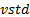, is relative to standard conditions defined as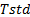= 70 ◦F and pstd = 14.7 psia. To determine the actual velocity,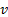act, in units of feet per minute, the equation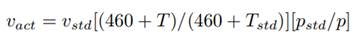must be applied, where T is in of and p is in psia. The accuracy of the reading on the velocimeter is 5.0 % or 5 ft/min, whichever is greater. The velocimeter also measures

air temperature with an accuracy of 1 ◦F. During an experiment, the measured air velocity is 400 ft/min and the temperature is 80 ◦F. The air pressure can be assumed to be at standard conditions. Determine (a) the actual air velocity, (b) the uncertainty in the actual air velocity,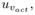and (c) the percent uncertainty in the actual air velocity.

Jul 26 2020 View more View LessSubscribe To Get Solution